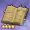# 「神石样本」研究笔记

2023-05-30更新

Leo丶莱茵

## 基础数据

• 「神石样本」研究笔记
• 航空
•  初始 10
•  强化+10 30
•  强化+11 32
• 炮击
•  初始 5
•  强化+10 15
•  强化+11 16
• 大型作战塞壬增伤 强化+11后获得此属性
• 强化+11 1%
• 技能 「神石样本」研究笔记
• 描述 进入战斗时，使自身对META舰船造成的伤害提升5%
• 装备参数
• 所属阵营 重樱
• 适用舰种
驱逐
轻巡
重巡
超巡
战巡
战列
轻航
航母
航战
潜母
重炮
潜艇
维修
运输
风帆

整装获取 其他：智者与蜘蛛## 装备导航

鱼雷 • 星云舞裙  • 引力舞鞋  • 闪耀之歌  • 活力之歌  • 炽烈之歌  • 改良声呐  • 基础声呐  • 维修工具  • 陀螺仪  • 改良锅炉  • 灭火器  • 海军迷彩  • 液压舵机
舰炮 • 超重弹  • 珍珠之泪  • SG雷达  • 雪顶之梦  • 火控雷达  • 对空雷达
舰炮 • 海大黄蜂  • 海怒  • 海毒牙  • 海喷火  • 海飓风  • 海斗士 • 飞龙  • 火冠  • 火把  • 梭鱼  • 青花鱼  • 剑鱼 • 萤火虫  • 海燕  • 贼鸥 • 小王冠  • 归航信标 • 刺猬弹
舰炮 • 紫电改二  • 烈风 • 流星  • 天山改  • 天山 • 彗星 • Z旗  • 「神石样本」研究笔记  • 刺绣锦囊 • 晴岚  • 强风  • 瑞云
舰炮 • 梅之语  • 四神之印 • 上游-1
舰炮 • 海魂迷彩
舰炮 • 天使之羽
舰炮 • 帆索组件
设备 • 智慧模块  • 晃悠悠
设备 • 鮟鱇肝  • 玉米灯笼  • 组徽
设备 • 心之钥匙  • 觉醒宝珠
设备 • 偶像手环
设备 • 煌翼炎龙  • 征战巨坦 • 木龙雕塑
设备 • 形意口琴  • 泡云弹车  • 创世之槌  • Ｎ／Ａ  • 默示录  • 万灵药剂  • 涡旋风精  • 震耳雷球  • 结晶冰精  • 炙烈炎烧
通常 • 苦无  • 短剑  • 权杖  • 猎弓  • 轻弩  • 指挥刀  • 骑枪  • 大剑  • 手弩  • 铁剑  • 单手锤  • 双剑 • 莱伯君  • 全开标枪  • 摇曳悠然 • 幻想之环  • 皮罗什基 • 魅力四射  • 不再哭泣 • 鸾凤流丽 • 烤白薯  • 马卡龙  • 帕帕纳西  • 甜馅馒头  • 公主蛋糕 • 监禁程序  • 龙的饰品  • 演出棒  • 怪兽种子  • 戴拿飞翼 • 百合长老  • 百花盛开  • 幽兰爪  • 爱瑟尔剑  • 暗夜星矢  • 闪耀梦想